Module 18 - Antiderivatives as Indefinite Integrals and Differential Equations

Introduction | Lesson 1 | Lesson 2 | Lesson 3 | Lesson 4 | Self-Test

Lesson 18.3: Solving Differential Equations Analytically

This lesson investigates finding analytical solutions to differential equations by using the TI-89's deSolve command and explores finding solutions to initial-value problems.

An equation containing a derivative such as y' = 2x, where y' denotes the derivative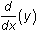, is called a differential equation. Such equations occur often in real-world applications.

Finding Solutions to Differential Equations

A solution to a differential equation is a function that satisfies the differential equation and there may be more than one solution. Each of the functions shown below is a solution to the differential equation y' = 2x because each makes the differential equation true.

y1 = x2

y2 = x2 + 1

y3 = x2 – 3

These particular solutions, as well as all other solutions to the differential equation y' = 2x, can be described by the function

y = x2 + C

where C represents any real number. This family of functions is called the general solution to the differential equation.

Using the deSolve Command

The TI-89 deSolve command can be used to find general solutions to simple differential equations like y' = 2x and to more complicated differential equations as well.

Solve y' = 2x by following the procedure below.

• Go to the Home Screen and execute NewProb
• Open the Calc menu by pressing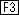• Scroll down the menu and highlight "C:deSolve(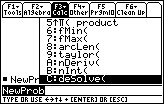• Paste this command to the Edit Line by pressing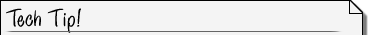deSolve Syntax The syntax for deSolve is deSolve(differential equation, independentVar, dependentVar)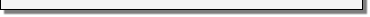• Execute deSolve(y'=2x,x,y)
• Note thatis above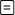.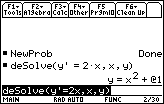Symbols for Arbitrary Constants The TI-89 uses the symbol @1 in the general solution above to represent the arbitrary constant C.The solution may be verified by substituting the result into the original differential equation.18.3.1 Use the deSolve command to find the general solution to the differential equation

y' = ex sin xSubsequent Arbitrary Constants The TI-89 used the symbol @2 for C in the result of Question 18.3.1 because it is the second arbitrary constant found since NewProb was last executed.18.3.2 Use deSolve to find the general solution to the differential equation

y' = y

Solving Initial-Value Problems

Solve the differential equation y' = 2x with the condition that y(0) = 1, that is, y = 1 when x = 0. The condition y(0) = 1 is called an initial condition and a differential equation with an initial condition is called an initial-value problem.

Solve the initial-value problem y' = 2x and y(0) = 1.

• Execute NewProb
• Execute deSolve(y'=2x and y(0)=1,x,y)
• Recall that "and " is found in the catalog.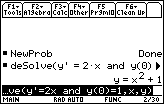The solution to an initial-value problem is called a particular solution to the differential equation. The initial condition selects a particular solution from the family of general solutions.

18.3.3 Solve the initial-value problem y' = 2x and y(2) = 1.Using the Multiplication Key in Differential Equations Do not use implied multiplication between a variable and an expression. For example, enter y = y*int(x), not y = yint(x).18.3.4 Solve the initial-value problem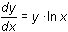and y(1) = 3. Don't forget to insert a multiplication sign between y and ln(x).